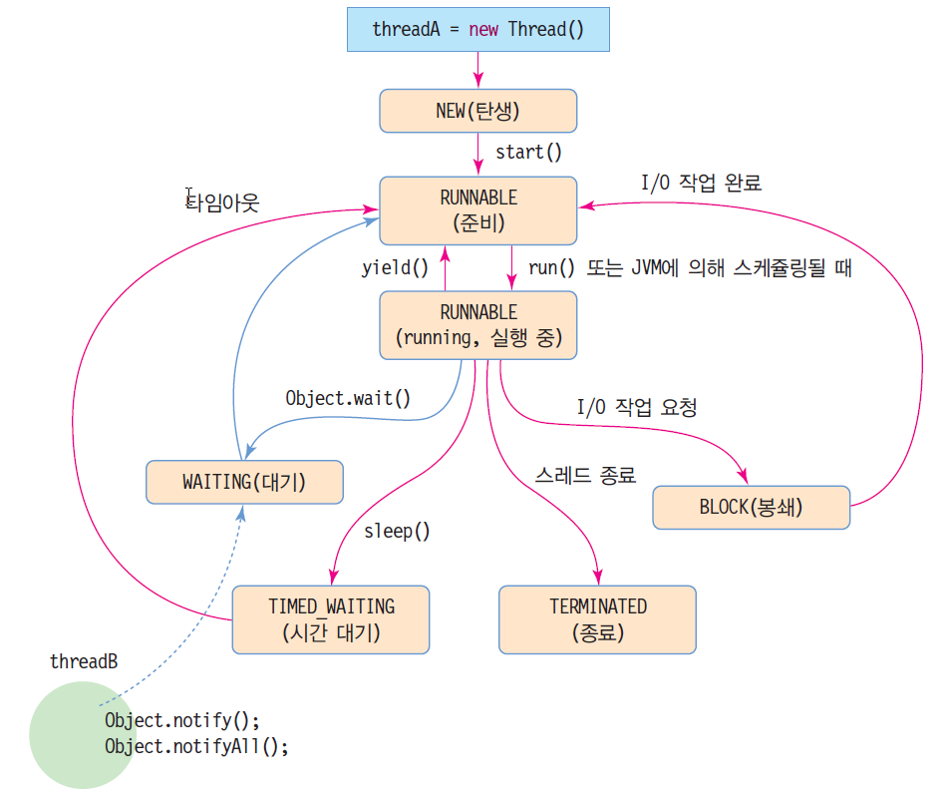### SWING

Java Layout Manager https://dis.dankook.ac.kr/lectures/java20/2020/05/31/java-layout-manager/
Java Swing Component https://dis.dankook.ac.kr/lectures/java20/2020/05/31/java-swing-component/
5 Ways to Implement Event Listener https://dis.dankook.ac.kr/lectures/java20/2020/05/31/5-ways-to-implement-event-listener/
ChangeListener, KeyListener, ActionListener https://dis.dankook.ac.kr/lectures/java20/2020/05/31/changelistener-keylistener-actionlistener/
Metric Converter (combobox ItemListener) https://dis.dankook.ac.kr/lectures/java20/2020/06/01/metric-converter/

### Lab4

Lab4 프로젝트 디렉토리 안에 모든 파일(src/*.java & bin/*.class)와 보고서 (3~4장)를 넣고 JAVA20-2_Lab4_학번_이름.zip 압축한 후 제출 (due by 10/12)

java2-lab4 (lab4-template을 이러닝에서 받아서 사용하세요.)

week6-lab4 동영상을 참고하세요.

### Java Thread yield() vs join() vs sleep()

We can prevent a thread from execution by using any of the 3 methods of Thread class:

• yield()
• join()
• sleep()

yield() method pauses the currently executing thread temporarily for giving a chance to the remaining waiting threads of the same priority to execute. If there is no waiting thread or all the waiting threads have a lower priority then the same thread will continue its execution. The yielded thread when it will get the chance for execution is decided by the thread scheduler whose behavior is vendor dependent (i.e., yield() can only make a heuristic attempt to suspend the execution of the current thread with no guarantee of when will it be scheduled back).

join() The current thread can invoke join() on any other thread which makes the current thread wait for the other thread to die before proceeding. If any executing thread t1 calls join() on t2 (i.e, t2.join()) immediately t1 will enter into waiting state until t2 completes its execution.

sleep() method can force the scheduler to suspend the execution of the current thread for a specified period of time as its parameter.### WeatherIndex

``````
// 체감온도값 = 13.12 + 0.6215*T - 11.37 * V^0.16 + 0.3965 * V^0.16 * T [ T: 기온(섭씨), V : 풍속(km/h) ]
public static double calculate(double T, double W) {
double V = fromMStoKMH(W);
double value = 0.0;
if (V > 4.8) {
value = 13.12 + 0.6215*T - 11.37 * Math.pow(V, 0.16) + 0.3965 * Math.pow(V, 0.16) * T;
if (value > T) {
value = T;
}
}
else {
value = T;
}
value = Math.round(value);
return value;
}
``````
``````
// 열지수값 = -42.379 + (2.04901523*F) + (10.14333127*R) - (0.22475541*F*R) - (0.00683770*F*F) - (0.05481717*R*R) + (0.00122874*F*F*R) + (0.00085282*F*R*R) - (0.00000199*F*F*R*R) [F: 화씨온도, R: 상대습도]
public static double calculate(double T, double R) {
double F = fromCelsiusToFahrenheit(T);
double value = -42.379 + (2.04901523*F) + (10.14333127*R) - (0.22475541*F*R) - (0.00683770*F*F) - (0.05481717*R*R) + (0.00122874*F*F*R) + (0.00085282*F*R*R) - (0.00000199*F*F*R*R);
if (R < 13.0 && F >= 80.0 && F <= 112.0) { adj = 0.25 * (13.0 - R) * Math.sqrt((17.0 - Math.abs(F - 95.0)) / 17.0); } if (R > 85.0 && F >= 80.0 && F <= 87) {
adj = (R - 85.0)/10.0 * (87.0 - F) / 5.0;
}
if (F < 80.0) {
value = F;
}
value = fromFahrenheitToCelsius(value); // (celsius)
value = Math.round(value * 10) / 10.0; // 소수점 첫째자리 반올림
return value;
}
``````
``````
// 부패지수값 = (RH - 65)/14 * (1.054^T) [RH: 상대습도 (%), T: 기온 (섭씨)]
public static double calculate(double T, double RH) {
double value = (RH - 65.0)/14.0 * Math.pow(1.054, T);
value = Math.round(value * 100) / 100.0; // 소수점 두째자리 반올림

return value;
}
``````

### Lab3

Lab3 프로젝트 디렉토리 안에 모든 파일(src/*.java & bin/*.class)와 보고서 (3~4장)를 넣고 JAVA20-2_Lab3_학번_이름.zip 압축한 후 제출 (due by 10/5)

java2-lab3 (lab3-template을 이러닝에서 받아서 사용하세요.)

week5-lab3 동영상을 참고하세요.

### Lab2

Lab2 프로젝트 디렉토리 안에 모든 파일(src/*.java & bin/*.class)와 보고서 (3~4장)를 넣고 JAVA20-2_Lab2_학번_이름.zip 압축한 후 제출 (due by 9/29)
java2-lab2

week4-lab2 동영상을 참고하세요.